Note

This page is a reference documentation. It only explains the function signature, and not how to use it. Please refer to the user guide for the big picture.

# nilearn.glm.first_level.make_first_level_design_matrix#

Generate a design matrix from the input parameters

Parameters:
frame_timesarray of shape (n_frames,)

The timing of acquisition of the scans in seconds.

eventsDataFrame instance, optional
Events data that describes the experimental paradigm.
The DataFrame instance might have these keys:
‘onset’: column to specify the start time of each events in

seconds. An error is raised if this key is missing.

‘trial_type’: column to specify per-event experimental conditions

identifier. If missing each event are labelled ‘dummy’ and considered to form a unique condition.

‘duration’: column to specify the duration of each events in

seconds. If missing the duration of each events is set to zero.

‘modulation’: column to specify the amplitude of each

events. If missing the default is set to ones(n_events).

An experimental paradigm is valid if it has an ‘onset’ key and a ‘duration’ key. If these keys are missing an error will be raised. For the others keys a warning will be displayed. Particular attention should be given to the ‘trial_type’ key which defines the different conditions in the experimental paradigm.

hrf_model`str`, function, list of functions, or None

This parameter defines the HRF model to be used. It can be a string if you are passing the name of a model implemented in Nilearn. Valid names are:

It can also be a custom model. In this case, a function should be provided for each regressor. Each function should behave as the other models implemented within Nilearn. That is, it should take both t_r and oversampling as inputs and return a sample numpy array of appropriate shape.

Note

It is expected that spm standard and glover models would not yield large differences in most cases.

Note

In case of glover and spm models, the derived regressors are orthogonalized wrt the main one.

drift_model{‘cosine’, ‘polynomial’, None}, optional

Specifies the desired drift model. Default=’cosine’.

high_passfloat, optional

High-pass frequency in case of a cosine model (in Hz). Default=0.01.

drift_orderint, optional

Order of the drift model (in case it is polynomial). Default=1.

fir_delaysarray of shape(n_onsets) or list, optional

In case of FIR design, yields the array of delays used in the FIR model (in scans). Default=.

additional user-supplied regressors, e.g. data driven noise regressors or seed based regressors.

If None, while add_regs was provided, these will be termed ‘reg_i’, i = 0..n_add_reg - 1 If add_regs is a DataFrame, the corresponding column names are used and add_reg_names is ignored.

min_onsetfloat, optional

Minimal onset relative to frame_times (in seconds) events that start before frame_times + min_onset are not considered. Default=-24.

oversamplingint, optional

Oversampling factor used in temporal convolutions. Default=50.

Returns:
design_matrixDataFrame instance,

holding the computed design matrix, the index being the frames_times and each column a regressor.

## Examples using `nilearn.glm.first_level.make_first_level_design_matrix`#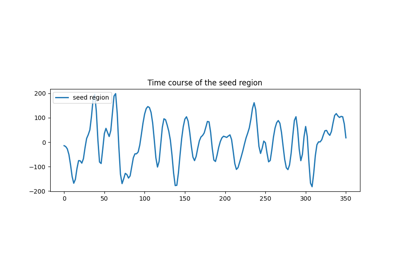Default Mode Network extraction of ADHD dataset

Default Mode Network extraction of ADHD dataset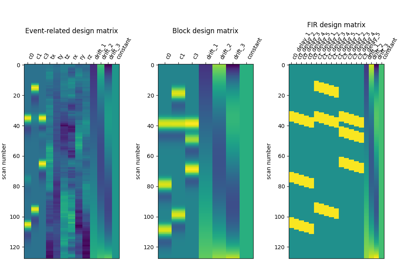Examples of design matrices

Examples of design matrices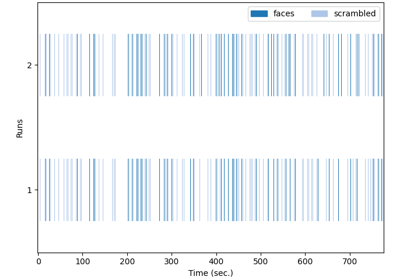Single-subject data (two sessions) in native space

Single-subject data (two sessions) in native space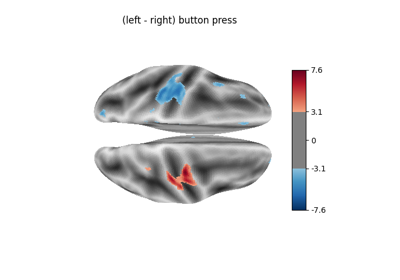Example of surface-based first-level analysis

Example of surface-based first-level analysis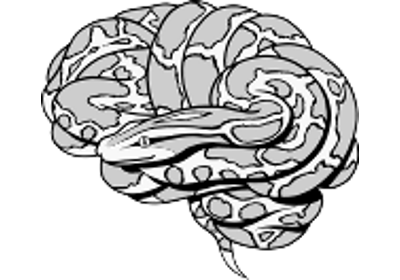Surface-based dataset first and second level analysis of a dataset

Surface-based dataset first and second level analysis of a dataset